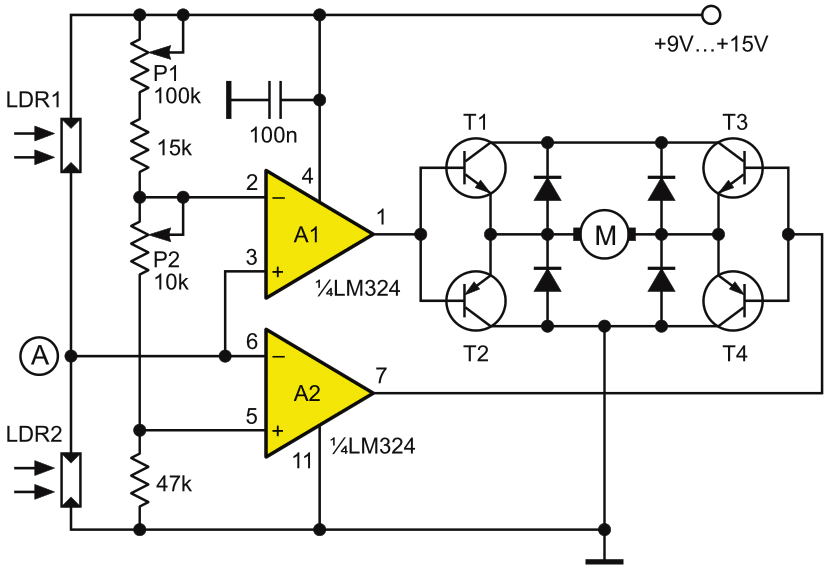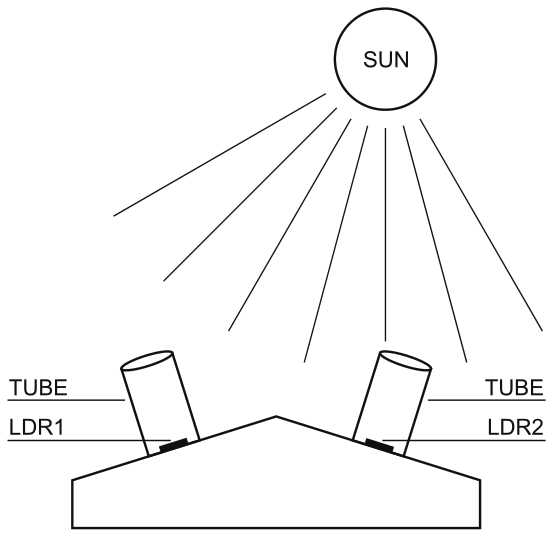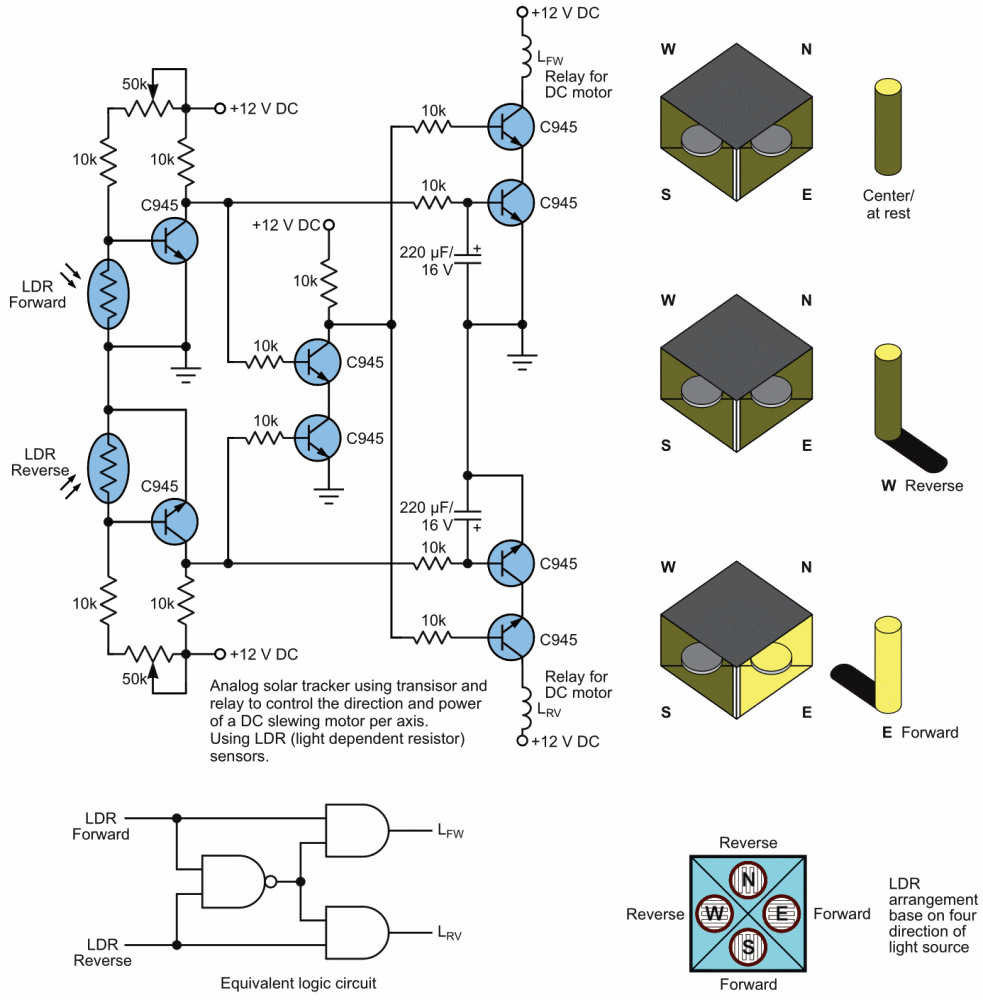# DIY solar tracker system

The solar panels are operating at optimal parameters when they are at the perfect right angle to the sun. Unfortunately this is accomplished only if solar panels are rotated by the sun. This is the purpose of this diy solar tracker system or if you have an Arduino board then you can build this one that uses a servo.

The solar tracker circuit (Figure 1) uses a window comparator to maintain the motor in a idle state as long as the two LDRs are under the same illumination level. In this case, half the voltage is applied to the noninverting input of A1 and to the inverting input of A1.Figure 1. Solar tracker system schematic.

## Solar tracker system

Solar tracking components:

• T1, T3 = BD239, BD139
• T2, T4 = BD240, BD140
• A1, A2 = 1/2 of LM324
• Diodes = 1N4001

When the sun position is changing so does the illumination level on the LDRs and the input voltage for the window comparator is no longer half of the supply voltage thereby the output of the comparator generates information for the motor that rotates the panels for tracking the sun.

## DIY sun tracking sensor

The sensor design is shown in Figure 2. P1 and P2 are adjusted in such way that the motor stands still when the LDRs get the same amount of solar light. If less light reaches LDR2 than LDR1, the voltage in point A increases to more than half of the power supply voltage. As a result the output of A1 is HIGH and T1 and T4 transistors conduct. In this situation the motor is starting.Figure 2. DIY sun tracking sensor.

If the angle of the solar light is changing again and the voltage in point A decreases at less than power supply voltage, the output of A2 goes HIGH and T3 and T2 transistors conduct. As a result the motor is rotating in opposite direction.

For solar panels control is best to use small motors with a suitable voltage and a maximum working current of 300 mA. This solar tracker system is used for tracking the sun only in one plane, the horizontal one. If you want to track the solar light in the vertical plane you need to build a separate sun tracker circuit.

## Analog solar panel tracker circuit by Bien FallariaFigure 3. Analog solar tracker circuit schematic by Bien.

This is a simple and practical analog solar panel tracker circuit. Using four LDR (light dependent resistor) as a sensor in detecting the light source arranged as illustrated in Figure 3. When the light hit the LDR in a certain position, it will activate the circuit and trigger the relay to turn the slewing motor in the right direction until the sensor is fully shaded under its cover stopping the motor to its rest condition.

electroschematics.com